#### Output Data Sets

##### OUTLIMITS= Data Set

The OUTLIMITS= data set saves the control limit parameters. The following variables can be saved:

Variable

Description

_ALPHA_

probability () of exceeding limits

_INDEX_

optional identifier for the control limits specified with the OUTINDEX= option

_LIMITN_

sample size associated with the control limits

_MEAN_

process mean (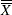or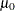)

_SIGMAS_

multiple (k) of standard error of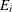_STDDEV_

process standard deviation (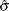or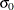)

_SUBGRP_

subgroup-variable specified in the EWMACHART statement

_TYPE_

type (estimate or standard value) of _MEAN_ and _STDDEV_

_VAR_

process specified in the EWMACHART statement

_WEIGHT_

weight (r) assigned to most recent subgroup mean in computation of EWMA

The OUTLIMITS= data set does not contain the control limits; instead, it contains control limit parameters that can be used to recompute the control limits.

Notes:

1. If the control limits vary with subgroup sample size, the special missing value V is assigned to the variable _LIMITN_.

2. If the limits are defined in terms of a multiple k of the standard error of, the value of _ALPHA_ is computed as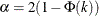, where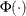is the standard normal distribution function.

3. If the limits are probability limits, the value of _SIGMAS_ is computed as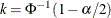, where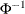is the inverse standard normal distribution function.

4. Optional BY variables are saved in the OUTLIMITS= data set.

The OUTLIMITS= data set contains one observation for each process specified in the EWMACHART statement.

You can use OUTLIMITS= data sets

• to keep a permanent record of the control limit parameters

• to write reports. You may prefer to use OUTTABLE= data sets for this purpose.

• as LIMITS= data sets in subsequent runs of PROC MACONTROL

For an example of an OUTLIMITS= data set, see the section Saving Control Limit Parameters.

##### OUTHISTORY= Data Set

The OUTHISTORY= data set saves subgroup summary statistics. The following variables can be saved:

• the subgroup-variable

• a subgroup mean variable named by process suffixed with X

• a subgroup standard deviation variable named by process suffixed with S

• a subgroup EWMA variable named by process suffixed with E

• a subgroup sample size variable named by process suffixed with N

Given a process name that contains 32 characters, the procedure first shortens the name to its first 16 characters and its last 15 characters, and then it adds the suffix.

Subgroup summary variables are created for each process specified in the EWMACHART statement. For example, consider the following statements:

proc macontrol data=Clips;
ewmachart (Gap YieldStrength)*Day /
weight     = 0.2
outhistory = Cliphist;
run;


The data set Cliphist would contain nine variables named Day, GapX, GapS, GapE, GapN, YieldStrengthX, YieldStrengthS, YieldStrengthE, and YieldStrengthN.

Additionally, the following variables, if specified, are included:

• BY variables

• block-variables

• symbol-variable

• ID variables

• _PHASE_ (if the OUTPHASE= option is specified)

For an example of an OUTHISTORY= data set, see the section Saving Summary Statistics.

##### OUTTABLE= Data Set

The OUTTABLE= data set saves subgroup summary statistics, control limits, and related information. The following variables can be saved:

Variable

Description

_ALPHA_

probability () of exceeding control limits

_EXLIM_

control limit exceeded on EWMA chart

_EWMA_

exponentially weighted moving average

_LCLE_

lower control limit for EWMA

_LIMITN_

nominal sample size associated with the control limits

_MEAN_

process mean

_SIGMAS_

multiple (k) of the standard error associated with control limits

subgroup

values of the subgroup variable

_SUBN_

subgroup sample size

_SUBS_

subgroup standard deviation

_SUBX_

subgroup mean

_UCLE_

upper control limit for EWMA

_VAR_

process specified in the EWMACHART statement

_WEIGHT_

weight (r) assigned to most recent subgroup mean in computation of EWMA

In addition, the following variables, if specified, are included:

• BY variables

• block-variables

• ID variables

• _PHASE_ (if the READPHASES= option is specified)

• symbol-variable

Notes:

1. Either the variable _ALPHA_ or the variable _SIGMAS_ is saved depending on how the control limits are defined (with the ALPHA= or SIGMAS= options, respectively, or with the corresponding variables in a LIMITS= data set).

2. The variables _VAR_ and _EXLIM_ are character variables of length 8. The variable _PHASE_ is a character variable of length 48. All other variables are numeric.

For an example of an OUTTABLE= data set, see Saving Control Limit Parameters.